# Lucky Darts

Learn creating a Lucky Darts game using Windows App SDK with this Tutorial

Lucky Darts shows how you can create a darts game with a random chance of hitting the right spot on the dartboard using a control in a toolkit from NuGet using the Windows App SDK.

## Step 1

Follow Setup and Start on how to get Setup and Install what you need for Visual Studio 2022 and Windows App SDK.

In Windows 11 choose Start and then find or search for Visual Studio 2022 and then select it.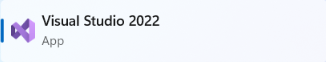Once Visual Studio 2022 has started select Create a new project.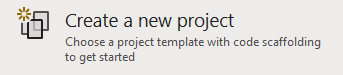Then choose the Blank App, Packages (WinUI in Desktop) and then select Next.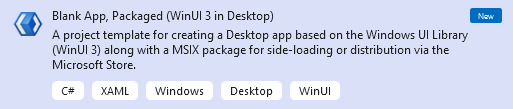After that in Configure your new project type in the Project name as LuckyDarts, then select a Location and then select Create to start a new Solution.## Step 2

Then in Visual Studio within Solution Explorer for the Solution, right click on the Project shown below the Solution and then select Manage NuGet Packages...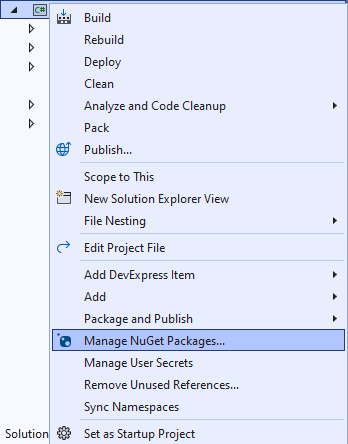## Step 3

Then in the NuGet Package Manager from the Browse tab search for Comentsys.Toolkit.WindowsAppSdk and then select Comentsys.Toolkit.WindowsAppSdk by Comentsys as indicated and select Install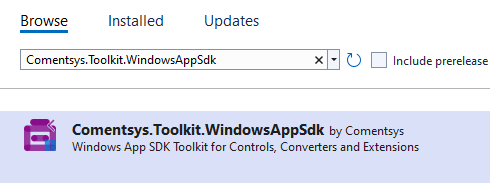This will add the package for Comentsys.Toolkit.WindowsAppSdk to your Project. If you get the Preview Changes screen saying Visual Studio is about to make changes to this solution. Click OK to proceed with the changes listed below. You can read the message and then select OK to Install the package, then you can close the tab for Nuget: LuckyDarts by selecting the x next to it.

## Step 4

Then in Visual Studio within Solution Explorer for the Solution, right click on the Project shown below the Solution and then select Add then New Item…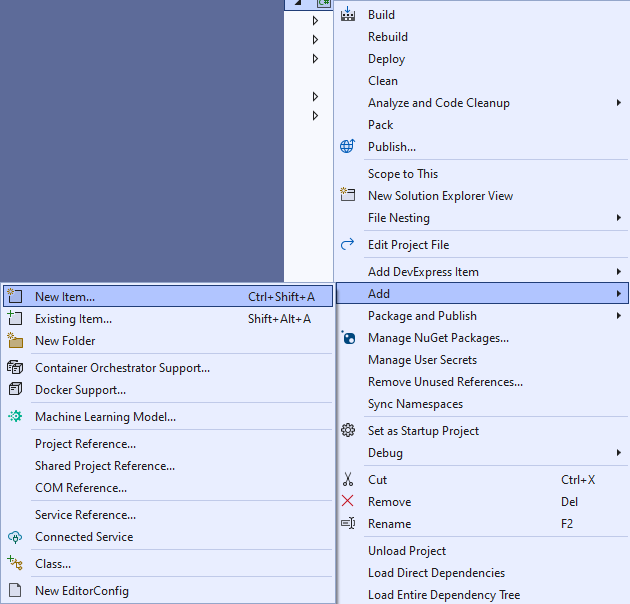## Step 5

Then in Add New Item from the C# Items list, select Code and then select Code File from the list next to this, then type in the name of Library.cs and then Click on Add.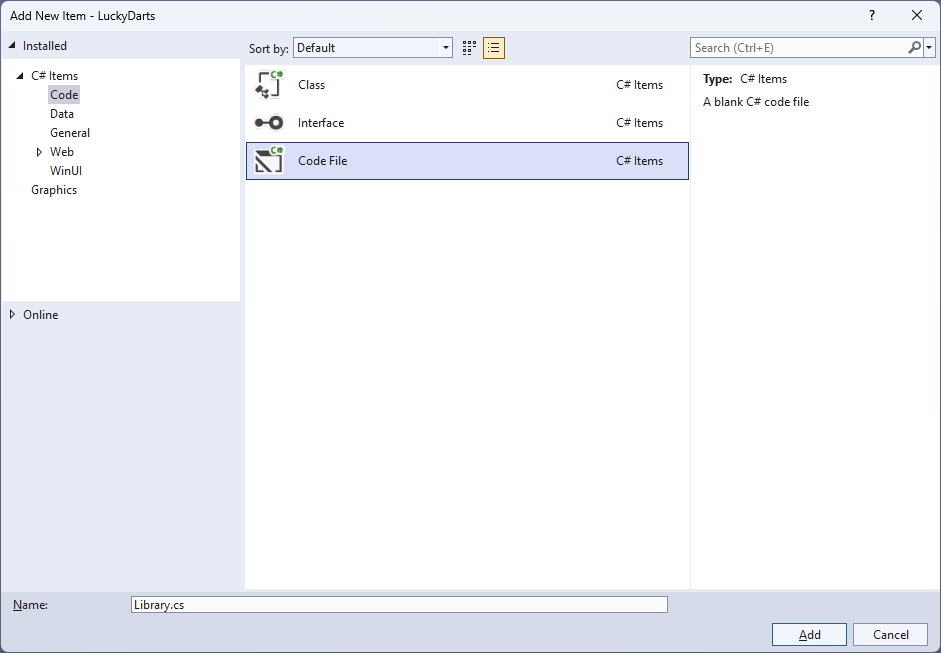## Step 6

You will now be in the View for the Code of Library.cs, within this type the following Code:

``````
using Comentsys.Toolkit.WindowsAppSdk;
using Microsoft.UI;
using Microsoft.UI.Xaml;
using Microsoft.UI.Xaml.Controls;
using Microsoft.UI.Xaml.Input;
using Microsoft.UI.Xaml.Media;
using Microsoft.UI.Xaml.Shapes;
using System;
using Windows.Foundation;
using Windows.UI;

public class Library
{
private const string title = "Lucky Darts";
private const int radius = 200;
private const int circle = 360;
private const int triple = 250;
private const int offset = 40;
private const int chance = 5;
private const int size = 500;
private const int dart = 10;
private const int bull = 20;
private const int ring = 10;
private const int font = 25;
private const int line = 2;
private static readonly int[] numbers =
{
20, 1, 18, 4, 13, 6, 10, 15, 2, 17,
3, 19, 7, 16, 8, 11, 14, 9, 12, 5
};
private static readonly double section =
circle / numbers.Length;
private readonly Random _random = new((int)DateTime.UtcNow.Ticks);

private int _score = 0;
private Dialog _dialog;
private Canvas _canvas;
private Piece _dart;

// Is Odd, Get Ellipse, Add Circle & Get Sector

// Add Dart & Get Number

// Get Score, Play & Add Board

// Layout & New

}
``````

The `Class` that has been defined in so far Library.cs has `using` for the package of `Comentsys.Toolkit.WindowsAppSdk` and others.

## Step 7

While still in the `Class` for Library.cs and after the Comment of `// Is Odd, Get Ellipse, Add Circle & Get Sector` type in the following Methods:

``````
private bool IsOdd(int value) =>
value % 2 != 0;

private Ellipse GetEllipse(double diameter, Color fill) => new()
{
Width = diameter,
Height = diameter,
StrokeThickness = line,
Fill = new SolidColorBrush(fill),
Stroke = new SolidColorBrush(Colors.WhiteSmoke)
};

private void AddCircle(Canvas canvas, double diameter, Color fill)
{
var circle = GetEllipse(diameter, fill);
Canvas.SetLeft(circle, (size - diameter) / 2);
Canvas.SetTop(circle, (size - diameter) / 2);
}

private Sector GetSector(double start, double finish,
double radius, double hole, Color fill)
{
Sector sector = new()
{
Hole = hole,
Start = start,
Finish = finish,
StrokeThickness = line,
Fill = new SolidColorBrush(fill),
Stroke = new SolidColorBrush(Colors.WhiteSmoke)
};
Canvas.SetLeft(sector, (size - radius * 2) / 2);
Canvas.SetTop(sector, (size - radius * 2) / 2);
return sector;
}
``````

`IsOdd` is used to determine if the number is odd or even, `GetEllipse` will get an `Ellipse` to be used with `AddCircle` and `GetSector` will use the Sector control from the toolkit and set it up as needed.

## Step 8

While still in the `Class` for Library.cs after the Comment of `// Add Section, Get Text & Add Text` type in the following Methods:

``````
private void AddSection(Canvas canvas, int index, double start)
{
var finish = section;
var sector = GetSector(start, finish, radius, bull,
IsOdd(index) ? Colors.Black : Colors.MintCream);
IsOdd(index) ? Colors.MediumSeaGreen : Colors.OrangeRed);
var tripleRing = GetSector(start, finish, triple / 2, triple / 2 - ring,
IsOdd(index) ? Colors.MediumSeaGreen : Colors.OrangeRed);
}

private TextBlock GetText(string value)
{
var text = new TextBlock()
{
Foreground = new SolidColorBrush(Colors.WhiteSmoke),
TextAlignment = TextAlignment.Center,
FontSize = font,
Text = value
};
text.Measure(new Size(
double.PositiveInfinity,
double.PositiveInfinity));
return text;
}

private void AddText(Canvas canvas, int index, double start)
{
var text = GetText(\$"{numbers[index]}");
double angle = start * Math.PI / (circle / 2);
double width = canvas.ActualWidth / 2;
double height = canvas.ActualHeight / 2;
double left = width + (width - font) * Math.Cos(angle)
- text.DesiredSize.Width / 2;
double top = height + (height - font) * Math.Sin(angle)
- text.DesiredSize.Height / 2;
Canvas.SetLeft(text, left);
Canvas.SetTop(text, top);
}
``````

`AddSection` will create a section of the dartboard using `GetSector` and `GetText` will create a `TextBlock` for the numbers to be used by `AddText` which positions the numbers on the dartboard.

## Step 9

While still in the `Class` for Library.cs after the Comment of `// Add Dart & Get Number` type the following Methods:

``````
{
_canvas.Children.Remove(_dart);
_dart = new Piece()
{
Width = dart,
Height = dart,
Name = nameof(_dart),
Fill = new SolidColorBrush(Colors.SlateGray),
Stroke = new SolidColorBrush(Colors.DarkGray)
};
Canvas.SetLeft(_dart, point.X - dart / 2);
Canvas.SetTop(_dart, point.Y - dart / 2);
}

private int GetNumber(double degrees) =>
degrees switch
{
>= 351 => 6,
>= 333 => 10,
>= 315 => 15,
>= 297 => 2,
>= 279 => 17,
>= 261 => 3,
>= 243 => 19,
>= 225 => 7,
>= 207 => 16,
>= 189 => 8,
>= 171 => 11,
>= 153 => 14,
>= 135 => 9,
>= 117 => 12,
>= 99 => 5,
>= 81 => 20,
>= 63 => 1,
>= 45 => 18,
>= 27 => 4,
>= 9 => 13,
_ => 6
};
``````

`AddDart` will position the dart on the dartboard with a given position and `GetNumber` will get the number of the dartboard based upon the angle passed in.

## Step 10

While still in the `Class` for Library.cs after the Comment of `// Get Score, Play & Add Board` type the following Methods:

``````
private int GetScore(Point point)
{
double x = point.X - size / 2;
double y = size / 2 - point.Y;
int degrees = (int)(radians * (circle / 2) / Math.PI);
degrees = degrees < 0 ? circle + degrees : degrees;
int number = GetNumber(degrees);
var length = (int)Math.Floor(Math.Sqrt(x * x + y * y));
return length switch
{
>= triple / 2 - ring and <= triple / 2 => number * 3,
>= bull - ring and <= bull => 25,
<= bull / 2 => 50,
_ => number
};
}

private void Play(Point point)
{
var x = _random.Next((int)point.X - offset, (int)point.X + offset);
var y = _random.Next((int)point.Y - offset, (int)point.Y + offset);
var hit = IsOdd(_random.Next(0, chance)) ? point : new Point(x, y);
var score = GetScore(hit);
_score += score;
_dialog.Show(\$"Scored {score}, Total {_score}");
}

private void AddBoard(Canvas canvas, double diameter)
{
var board = GetEllipse(diameter, Colors.Transparent);
board.Tapped += (object sender, TappedRoutedEventArgs e) =>
Play(e.GetPosition(canvas));
Canvas.SetLeft(board, (size - diameter) / 2);
Canvas.SetTop(board, (size - diameter) / 2);
}
``````

`GetScore` will work out the score of the position on the dartboard, taking into account the double ring, triple ring, outer bullseye and the inner bullseye. `Play` will position the dart on the dart board in a randomised location near where was selected and then the score will be updated accordingly and is used in `AddBoard` which will add a transparent `Ellipse` which will capture Events when it is Tapped.

## Step 11

While still in the `Class` for Library.cs after the Comment of `// Layout & New` type the following Methods:

``````
private void Layout(Grid grid)
{
grid.Children.Clear();
_canvas = new Canvas()
{
Width = size,
Height = size
};
var start = -(section / 2);
for (int index = 0; index < numbers.Length; index++)
{
AddText(_canvas, index, start + (section / 2) - (circle / 4));
start += section;
}
}

public void New(Grid grid)
{
_score = 0;
_dialog = new Dialog(grid.XamlRoot, title);
Layout(grid);
}
``````

`Layout` will create the look-and-feel of the dartboard and `New` will start a new game.

## Step 12

Within Solution Explorer for the Solution double-click on MainWindow.xaml to see the XAML for the Main Window.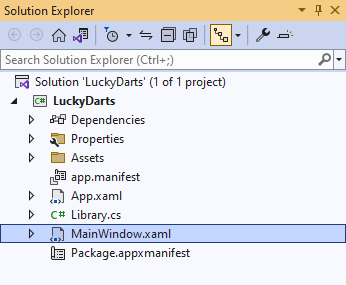## Step 13

In the XAML for MainWindow.xaml there will be some XAML for a `StackPanel`, this should be Removed:

``````
<StackPanel Orientation="Horizontal"
HorizontalAlignment="Center" VerticalAlignment="Center">
<Button x:Name="myButton" Click="myButton_Click">Click Me</Button>
</StackPanel>
``````

## Step 14

While still in the XAML for MainWindow.xaml above `</Window>`, type in the following XAML:

``````
<Grid>
<Viewbox>
<Grid Margin="50" Name="Display"
HorizontalAlignment="Center"
</Viewbox>
<CommandBar VerticalAlignment="Bottom">
<AppBarButton Icon="Page2" Label="New" Click="New"/>
</CommandBar>
</Grid>
``````

This XAML contains a `Grid` with a `Viewbox` which will Scale a `Grid`. It has a `Loaded` event handler for `New` which is also shared by the`AppBarButton`.

## Step 15

Within Solution Explorer for the Solution select the arrow next to MainWindow.xaml then double-click on MainWindow.xaml.cs to see the Code for the Main Window.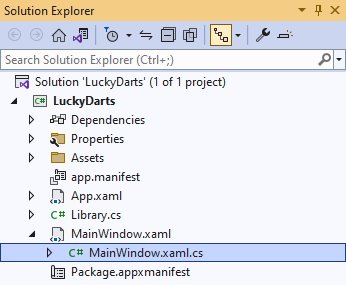## Step 16

In the Code for `MainWindow.xaml.cs` there be a Method of `myButton_Click(...)` this should be Removed by removing the following:

``````
private void myButton_Click(object sender, RoutedEventArgs e)
{
myButton.Content = "Clicked";
}
``````

## Step 17

Once `myButton_Click(...)` has been removed, within the Constructor of `public MainWindow() { ... }` and below the line of `this.InitializeComponent();` type in the following Code:

``````
private readonly Library _library = new();

private void New(object sender, RoutedEventArgs e) =>
_library.New(Display);
``````

Here an Instance of the `Class` of `Library` is created then below this is the Method of `New` that will be used with Event Handler from the XAML, this Method uses Arrow Syntax with the `=>` for an Expression Body which is useful when a Method only has one line.

## Step 18

That completes the Windows App SDK application. In Visual Studio 2022 from the Toolbar select LuckyDarts (Package) to Start the application.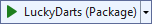## Step 19

Once running you can then select anywhere on the dartboard to try and see if you can get that score but there's a random chance you won't hit that spot and see what score you can get or select New to restart the game.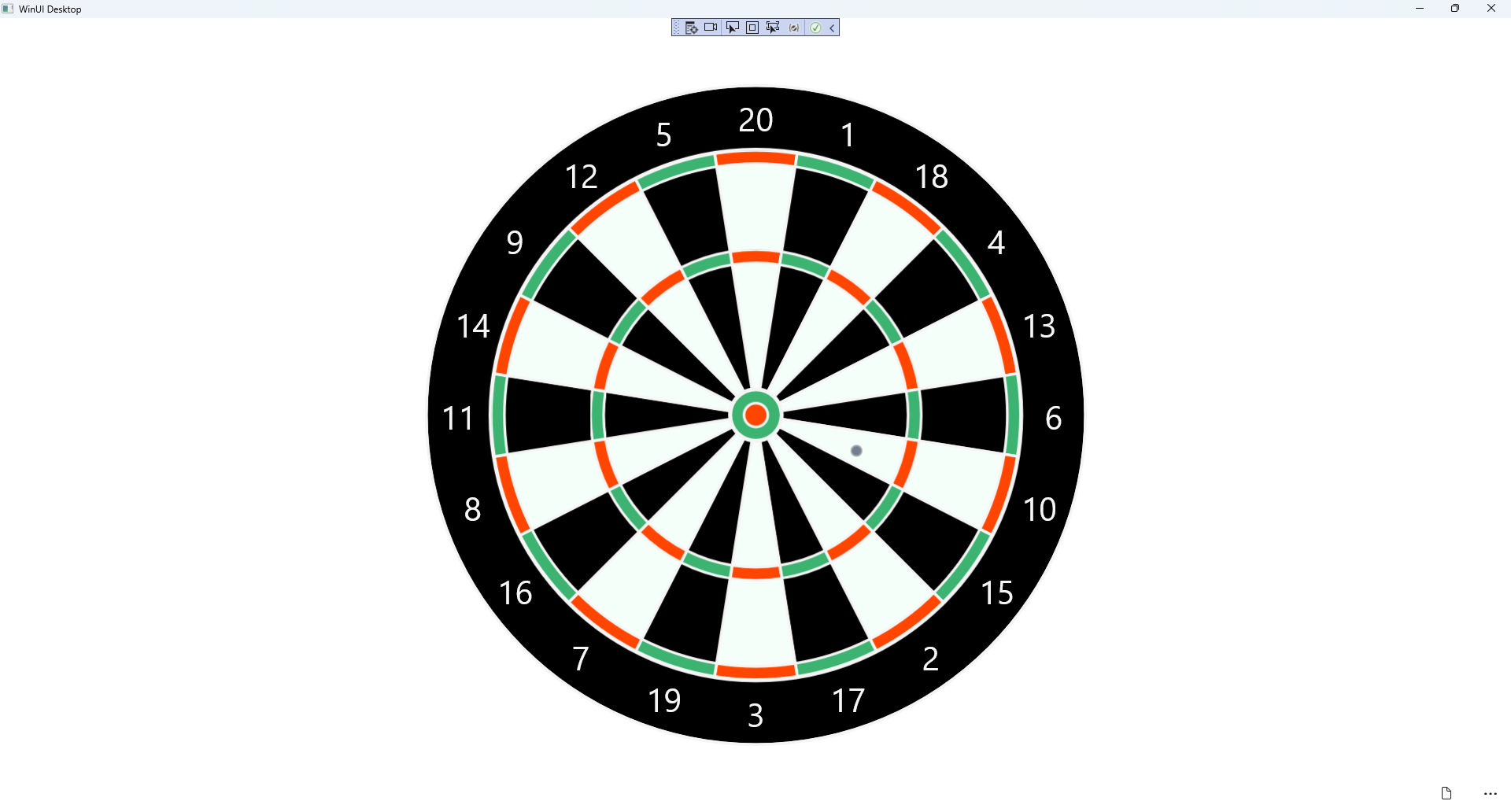## Step 20

To Exit the Windows App SDK application, select the Close button from the top right of the application as that concludes this Tutorial for Windows App SDK from tutorialr.com!